Chapter 7. Energy and Chemistry

# Stoichiometry Calculations Using Enthalpy

Learning Objectives

1. Perform stoichiometry calculations using energy changes from thermochemical equations.

In Chapter 5 “Stoichiometry and the Mole”, we related quantities of one substance to another in a chemical equation by performing calculations that used the balanced chemical equation; the balanced chemical equation provided equivalences that we used to construct conversion factors. For example, observe the following balanced chemical equation: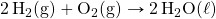In this equation, we recognize the following equivalences: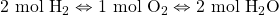Where ⇔ is the mathematical symbol for “is equivalent to.” In our thermochemical equation, however, we have another quantity — energy change: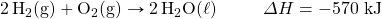This new quantity allows us to add another equivalence to our list: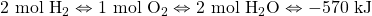That is, we can now add an energy amount to the equivalences — the enthalpy change of a balanced chemical reaction. This equivalence can also be used to construct conversion factors so that we can relate enthalpy change to amounts of substances reacted or produced.

Note that these equivalences address a concern. When an amount of energy is listed for a balanced chemical reaction, what amount(s) of reactants or products does it refer to? The answer is that relates to the number of moles of the substance as indicated by its coefficient in the balanced chemical reaction. Thus, 2 mol of H2 are related to −570 kJ, while 1 mol of O2 is related to −570 kJ. This is why the unit on the energy change is kJ, not kJ/mol.

For example, consider the following thermochemical equation: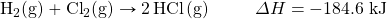The equivalences for this thermochemical equation are: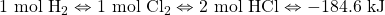Suppose we asked how much energy is given off when 8.22 mol of H2 react. We would construct a conversion factor between the number of moles of H2 and the energy given off, −184.6 kJ: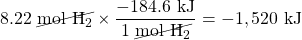The negative sign means that this much energy is given off.

Example 7.6

# Problem

Determine how much energy is given off when 222.4 g of N2 reacts in the following thermochemical equation: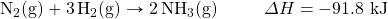## Solution

The balanced thermochemical equation relates the energy change to moles, not grams, so we first convert the amount of N2 to moles and then use the thermochemical equation to determine the energy change: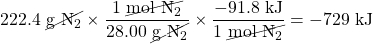# Test Yourself

Determine how much heat is given off when 1.00 g of H2 reacts in the following thermochemical equation:−15.1 kJ

Like any stoichiometric quantity, we can start with energy and determine an amount, rather than the other way around.

Example 7.7

# Problem

Determine what mass of NO can be made if 558 kJ of energy are supplied, given the following thermochemical equation: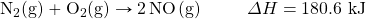## Solution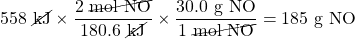# Test Yourself

Given the following equation, how many grams of N2 will react if 100.0 kJ of energy are supplied?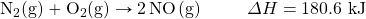15.5 g

# Chemistry Is Everywhere: Welding with Chemical Reactions

One very energetic reaction is called the thermite reaction. Its classic reactants are aluminum metal and iron(III) oxide; the reaction produces iron metal and aluminum oxide: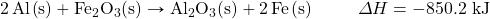When properly done, the reaction gives off so much energy that the iron product comes off as a liquid. (Iron normally melts at 1,536°C.) If carefully directed, the liquid iron can fill spaces between two or more metal parts and, after it quickly cools, can weld the metal parts together.

Thermite reactions are used for this purpose even today. For civilian purposes, they are used to re-weld broken locomotive axles that cannot be easily removed for repair. They are used to weld railroad tracks together. Thermite reactions can also be used to separate thin pieces of metal if, for whatever reason, a torch doesn’t work.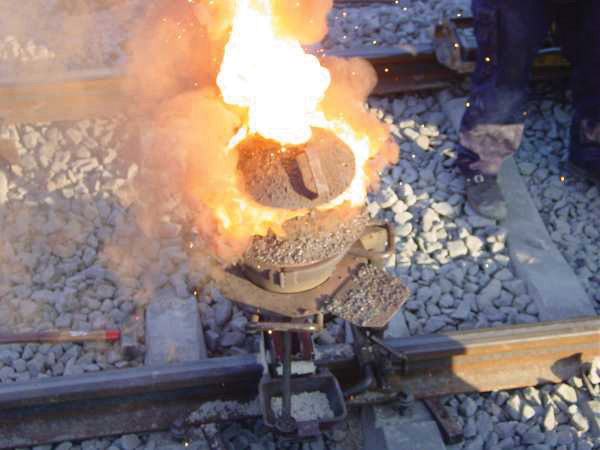Figure 7.2 “Thermite Reaction.” A small clay pot contains a thermite mixture. It is reacting at high temperature in the photo and will eventually produce molten metal to join the railroad tracks below it.

Thermite reactions are also used for military purposes. Thermite mixtures are frequently used with additional components as incendiary devices — devices that start fires. Thermite reactions are also useful in disabling enemy weapons: a piece of artillery doesn’t work so well when it has a hole melted into its barrel because of a thermite reaction!

Key Takeaways

• The energy change of a chemical reaction can be used in stoichiometry calculations.

Exercises

# Questions

1. Write the equivalences that this balanced thermochemical equation implies: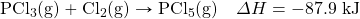2. Write the equivalences that this balanced thermochemical equation implies: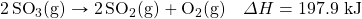3. How many kilojoules are given off when 17.8 mol of CH4(g) react in the following equation?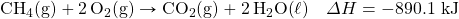4. How many kilojoules are absorbed when 0.772 mol of N2(g) reacts in the following equation?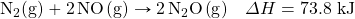5. How many kilojoules are absorbed when 23.09 mol of C6H6(ℓ) are formed in the following equation?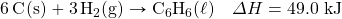6. How many kilojoules are given off when 8.32 mol of Mg react in the following equation?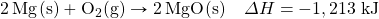7. Glucose (C6H12O6) is the main fuel metabolized in animal cells. How much energy is given off when 100.0 g of C6H12O6 react in the following equation?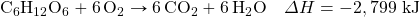8. How much energy is given off when 288 g of Fe are produced, given the following thermochemical equation?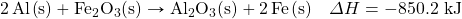9. How much energy is absorbed when 85.2 g of CO2 are reacted in the following thermochemical equation?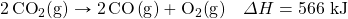10. How much energy is absorbed when 55.9 g of Na+(aq) are reacted in the following thermochemical equation?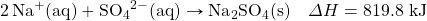11. NaHCO3 decomposes when exposed to heat. What mass of NaHCO3 is decomposed by 256 kJ, in the following thermochemical equation?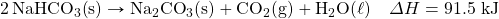12. HgO decomposes when exposed to heat. What mass of O2 can be made with 100.0 kJ, in the following thermochemical equation?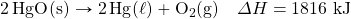13. What mass of SO3 is needed to generate 1,566 kJ in the following thermochemical equation?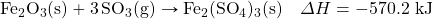14. What mass of HBr will be formed when 553 kJ of energy are given off in the following thermochemical equation?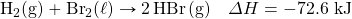1. 1 mol PCl3 ⇔ 1 mol Cl2 ⇔ 1 mol PCl5 ⇔ −87.9 kJ
1. 15,800 kJ
1. 1,130 kJ
1. 1,554 kJ
1. 548 kJ
1. 470 g
1. 6.60 × 102 g